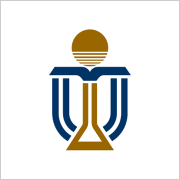## 課程信息

40,110 次近期查看

100% 在線

Knowledge of calculus, matrix algebra, differential equations and a computer programming language

### 您將學到的內容有

• MATLAB and Scientific Computing

• Root Finding and Numerical Matrix Algebra

• Numerical Solution of Ordinary and Partial Differential Equations

## 您將獲得的技能

Numerical AnalysisEngineering MathematicsMATLAB programming

100% 在線

Knowledge of calculus, matrix algebra, differential equations and a computer programming language

## 提供方1

## Scientific Computing

15 個視頻 （總計 108 分鐘）, 13 個閱讀材料, 11 個測驗
2

## Root Finding

12 個視頻 （總計 130 分鐘）, 8 個閱讀材料, 4 個測驗
3

## Matrix Algebra

13 個視頻 （總計 115 分鐘）, 9 個閱讀材料, 5 個測驗
4